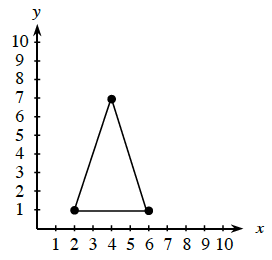### Home > CC2 > Chapter 1 > Lesson 1.2.7 > Problem1-127

1-127.

Locate the coordinates of the three highlighted points on the graph of the triangle at right and write them as ordered pairs $(x,y)$.To determine the x-coordinate of a point, find the horizontal distance from the origin.
To determine the y-coordinate of a point, find the vertical distance from the origin.

One ordered pair is $(2,1)$.

Don't forget to put the $x$-coordinate before the $y$-coordinate in your ordered pair. $(x,y)$